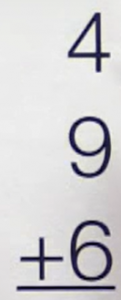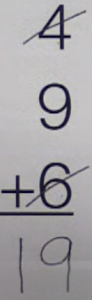# Lesson 5: Find the Tens to Avoid Counting One by One with worksheets

We are going to learn that there is more than one way to solve the problem. I’m going to show you two ways to solve the next problem, the hard way and the easy way.

Here we have the problem, four plus nine plus six.The hard way to solve this problem is to count one at a time. So a student might start with four and add nine more. So we have 5, 6, 7, 8, 9, 10, 11, 12, 13 plus six more gives us 14, 15, 16, 17, 18, 19.That is the correct answer, but it took excessively long.

Let’s learn an easier way.

Instead of counting one at a time, let’s use two strategies that we already know. Which are find the tens and ten in another.

So looking at these three numbers, which two numbers add up to ten? We know that four and six give you ten. So cross them off and say ten. Now ten plus nine more gives you 19.Notice how we did not have to count one by one.

Let’s take a look at the assignment.

In part one, students find the missing add ins that make a ten.

Look at part two.

Students use a ten and another strategy to add without having to count one by one.

Finally, part three. Students need to add without counting one by one. Find the two atoms that make a ten, and then use the ten and another strategy.

In the example, one and nine give you ten. So they’re crossed off. Ten plus seven give you 17.